Courses

# Linear Algebra (Advance Level) - 1

## 15 Questions MCQ Test Question Bank for GATE Computer Science Engineering | Linear Algebra (Advance Level) - 1

Description
This mock test of Linear Algebra (Advance Level) - 1 for GATE helps you for every GATE entrance exam. This contains 15 Multiple Choice Questions for GATE Linear Algebra (Advance Level) - 1 (mcq) to study with solutions a complete question bank. The solved questions answers in this Linear Algebra (Advance Level) - 1 quiz give you a good mix of easy questions and tough questions. GATE students definitely take this Linear Algebra (Advance Level) - 1 exercise for a better result in the exam. You can find other Linear Algebra (Advance Level) - 1 extra questions, long questions & short questions for GATE on EduRev as well by searching above.
QUESTION: 1

### The rank of the following ( n + 1 ) x ( n + 1) matrix, where a is a real number is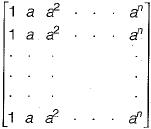Solution:

All the rows of the given matrix is same. So the matrix has only one independent row.
Rank of the matrix = Number of independent rows of the matrix.
∴ Rank of given matrix = 1

QUESTION: 2

### Let AX - B be a system of linear equations where A is an m x n matrix and b is a m x 1 column vector and X is an x 1 column vector of unknown. Which of the following is false?

Solution:

Following are the possibilities for a system of linear equations:
(i) If matrix A and augmented matrix [AB] have same rank, then the system has solution otherwise there is no solution.
(ii) If matrix A and augmented matrix [AB] have same rank which is equal to the number of variables, then the system has unique solution and if B is zero vector then the system have only a trivial solution.
(iii) If matrix A and matrix [AB] have same rank which is less than the number of variables, then the system has infinite solution.
Therefore, option (c) is false because if m - n and B is non-zero vector, then it is not necessary that system has a unique solution, because m is the number of equations (quantity) and not the number of linearly independent equations (quality).

QUESTION: 3

### The matrices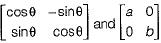commute under multiplication

Solution: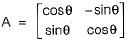and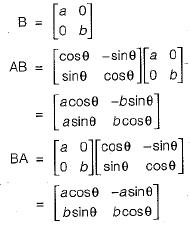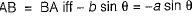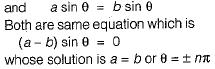QUESTION: 4

Consider the following set of equations:
x + 2y = 5
4x + 8y = 12
3x + 6y + 3z = 15
This set

Solution:

Set of equations is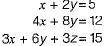Above set of equations can be written as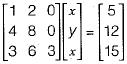Augmented matrix [AB] is given as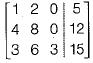Performing gauss-Elimination on the above matrix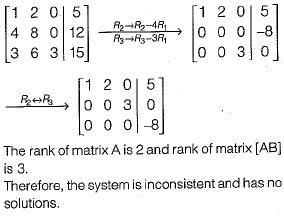QUESTION: 5

An n x n array v is defined as follows: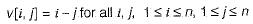The sum of the elements of the array v is

Solution:

The matrix V can be defined as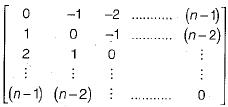So above is antisymmetric matrix and the sum of the elements of any antisymmetric matrix is 0.
OR
Alternate Method: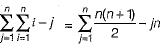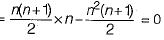QUESTION: 6

Consider the following statements:
S1: The sum of two singular n x n matrices may be non-singular
S2: The sum of two n x n non-singular matrices may be singular
Which of the following statements is correct?

Solution:

S1 is true
Consider two singular matrices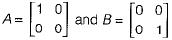Sum of A and B is given as
However (A + B) is a non-singuiar matrix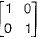So, S1 is true.
Now, consider two non-singuiar matrices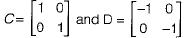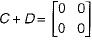However (C + D) is a singular matrix. So, S2 is also true.
Therefore, both S1 and S2 are true.

QUESTION: 7

Let A, B, C, D be n x n matrices, each with non-zero determinant, If ABCD = I, then B-1 is

Solution:

A, B, C, D is n x n matrix.
Given, ABCD = I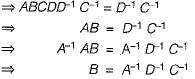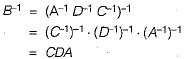QUESTION: 8

What values of x, y and z satisfy the following system of linear equations?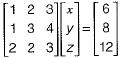Solution: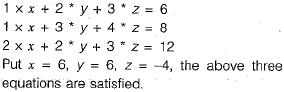QUESTION: 9

How many solutions does the following system of linear equations have?
- x + 5y = - 1
x - y = 2
x + 3y = 3

Solution: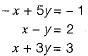The augmented matrix is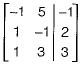Using gauss-efimination on above matrix we get,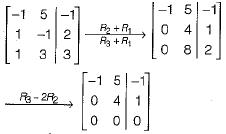Rank [A | B] - 2 (number of non zero rows in [A | B])
Rank [A ] = 2 (number of non zero row s in [A ])
Rank [A | B] = Rank [A] = 2 = number of variables
∴ Unique solution exists.

QUESTION: 10

Consider the following system of equations in three real variables x1, x2 and x3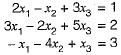This system of equations has

Solution:

The augmented matrix for the given system is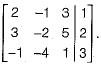Using gauss -elimination method on above matrix we get,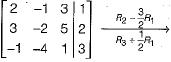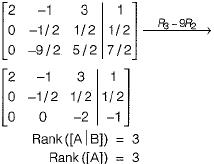Since Rank ([A | B]) = Rank ([A]) = number of variables, the system has unique solution.

QUESTION: 11

What are the eigenvalues of the following 2 x 2 matrix?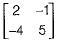Solution: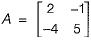The characteristic equation of this matrix is given by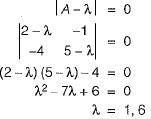∴ The eigen values of A are 1 and 6.

QUESTION: 12

F is an n x n real matrix, b is an n x 1 real vector. Suppose there are two n x 1 vectors, u and v such that u ≠ v and Fu = b, Fv = b.
Which one of the following statements is false?

Solution:

Given that Fu= b and Fv= b
If F is non singular, then it has a unique inverse.
Now, u = F-1 b and v = F-1 b
Since F-1 is unique u = v but it is given that u ≠ v .
This is a contradiction. So F must be singular.
This means that
(a) Determinate of F is zero is true. Also
(b) There are infinite number of solution to Fx = b is true since |F| = 0
(c) There is an X ≠ 0 such that FX = 0 is also true, since X has infinite number of solutions, including the X = 0 solution.
(d) F must have 2 identical rows is false, since a determinant may become zero, even if two identical columns are present. It is not necessary that 2 identical rows must be present for |F| to become zero.

QUESTION: 13

What are the eigenvalues of the matrix P given below: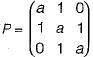Solution: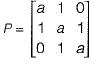Since P is a square matrix
Sum (eigen values) = Trace (P)
= a + a + a = 3a
Product of eigen values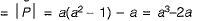Only choice (a) has sum of eigen values = 3a and product of eigen values = a3 - 2a.

QUESTION: 14

If the rank of a (5 x 6) matrix Q is 4, then which one of the following statements is correct?

Solution:

If rank of (5 x 6) matrix is 4, then surely it must have exactly 4 linearly independent rows as will as 4 linearly in dependent columns.

QUESTION: 15

The trace and determinant of a 2 x 2 matrix are known to be -2 and -35 respectively. It eigenvalues are

Solution:

Trace = Sum of principal diagonal element.